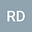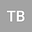Shell equations in terms of Günter’s derivatives, derived by the Г-convergence
••• Roland Duduchava,
• Tengiz BUCHUKURI
Roland Duduchava
The University of Georgia
Author ProfileTengiz BUCHUKURI
Tbilisi State University, A. Razmadze Mathematical Institute
Author Profile#### Peer review status:UNDER REVIEW

25 Feb 2020Submitted to Mathematical Methods in the Applied Sciences
01 Mar 2020Assigned to Editor
01 Mar 2020Submission Checks Completed
01 Mar 2020Reviewer(s) Assigned

## Abstract

A mixed boundary value problem for the L\’ame equation in a thin layer $\Omega^h:\cC\times[-h,h]$ around a surface $\cC$ with the Lipshitz boundary is investigated. The main goal is to find out what happens when the thickness of the layer tends to zero $h\to0$. To this end we reformulate BVP into an equivalent variational problem and prove that the energy functional has the $\Gamma$-limit being the energy functional on the mid-surface $\cC$. The corresponding BVP on $\cC$, considered as the $\Gamma$-limit of the initial BVP, is written in terms of G\”unter’s tangential derivatives on $\cC$ and represents a new form of the shell equation. It is shown that the Neumann boundary condition from the initial BVP on the upper and lower surfaces transforms into a right-hand side term of the basic equation of the limit BVP.